alonsoformula In Spanish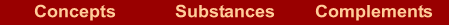What are they?  |  How are they named? If we know the formula  | If we know the name  |  Examples  |  Exercises

What are they?

They are compounds formed by hydrogen and a nonmetal, a chalcogen (group 16) or halogen (group 17), in which the hydrogen has an oxidation number +1.

In these compounds hydrogen is written to the left of the nonmetal.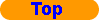How are they named?

The name is formed by two words. First word "hydrogen". Second word is the root of the nonmetal with a suffix "-ide".

Hydrogen NONMETAL-ide

These compounds are called hydracids because dissolve in water are acid dissolutions. It uses the subscript (aq) to indicate an aqueous solution.

In aqueous solution the name is formed by two words. First word a prefix "hydro-" connected to the root of the element with a suffix "-ic". Second word is the word "acid".

Hydro-NONMETAL-ic acidIf we know the formula

In the formula: Nonmetal is named with the suffix "-ide"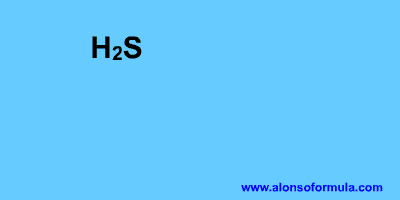In the formula: In aqueous solution the name is hydro-NONMETAL-ic acid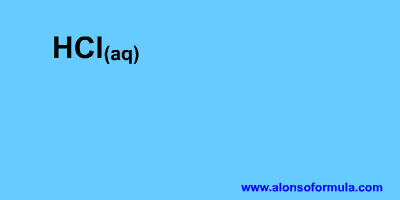If we know the name

In the name: The number of hydrogen matches up with the oxidation number of nonmetal.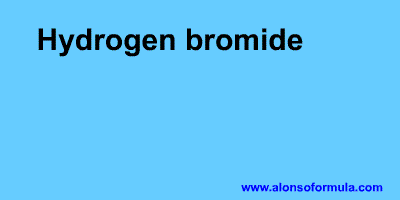In the name: The number of hydrogen matches up with the oxidation number of nonmetal.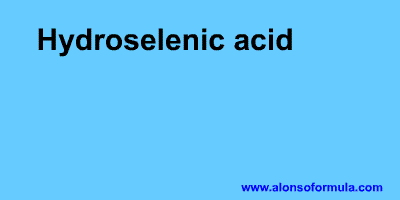Examples

In this group we include the HCN.

 Systematic name Aqueous solution name HF Hydrogen fluoride HF(aq) Hydrofluoric acid HCl Hydrogen chloride HCl(aq) Hydrochloric acid H2S Hydrogen sulfide H2S(aq) Hydrosulfuric acid H2Te Hydrogen telluride H2Te(aq) Hydrotelluric acid HCN Hydrogen cyanide HCN(aq) Hydrocyanic acidExercises

In Formulae you have an exercise to write the names of these substances and to check your results. You also have the answer to the exercise.

In Names you have an exercise to write the formulae for these substances and to check your results. You have to introduce the formulae without subscripts, for example for water = H2O. You also have the answer to the exercise.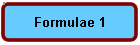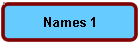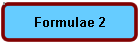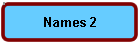Home | Introduction | Oxidation number | Mechanics | Kind of substances | Elementary substances | Metal oxides | Nonmetal oxides | Metal-nonmetal compounds | Nonmetal-nonmetal compounds | Metal hydrides | Hydracids | H with nonmetal | Hydroxides | Oxyacids | Oxysalts | Acid salts | Exercises | Periodic Table | Links
Author: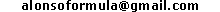WWW.ALONSOFORMULA.COM Formulación InorgánicaFormulación OrgánicaFormulación Inorgánica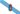Formulación OrgánicaFormulació Inorgánica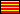Formulació OrgánicaEzorganikoaren Formulazioa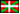Nomenclature of Inorganic Q.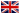Física y Química de ESOFísica e Química de ESOFQ de 1º de BachilleratoFQ de 1º de BacharelatoQuímica de 2º de BachilleratoPrácticas de Química